Trial ends in
JoVE Science Education
Aeronautical Engineering

A subscription to JoVE is required to view this content.
You will only be able to see the first 20 seconds.

Airfoil Behavior
##### Science Education (Aeronautical Engineering)
Real-time Flight Control: Embedded Sensor Calibration and Data Acquisition

# Airfoil Behavior: Pressure Distribution over a Clark Y-14 Wing

### Overview

Source: David Guo, College of Engineering, Technology, and Aeronautics (CETA), Southern New Hampshire University (SNHU), Manchester, New Hampshire

An airfoil is a 2-dimensional wing section that represents critical wing performance characteristics. The pressure distribution and lift coefficient are important parameters that characterize the behavior of airfoils. The pressure distribution is directly related to the lift generated by airfoils. A Clark Y-14 airfoil, which is used in this demonstration, has a thickness of 14% and is flat on the lower surface from 30% of chord length to the back.

Here we will demonstrate how the pressure distribution around an airfoil is measured using a wind tunnel. A Clark Y-14 airfoil model with 19 pressure ports is used to collect pressure data, which is used to estimate the lift coefficient.

### Principles

An airfoil develops lift at various angles of attack through lower gage pressures on the upper surface and higher gage pressures on the lower surface with respect to the pressure of the approaching air (free-stream pressure). If the shear forces parallel to the surface of the airfoil are neglected (typically their contributions to lift are small), then the total pressure force is the reason for the lift generated by the airfoil. Figure 1 shows a schematic of the pressure distribution over an airfoil.Figure 1. Pressure distribution over an airfoil.

The non-dimensional pressure coefficient, Cp, for an arbitrary point on the airfoil is defined as:(1)

where P is the absolute pressure, P is the undisturbed free-stream pressure, Pgage = P − P is the gage pressure, andis the dynamic pressure, which is based on the free-stream density, ρ, and airspeed, V.

The non-dimensional lift coefficient Cis similarly defined: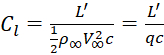(2)

where L' is the lift per unit span, and c is the chord length of the airfoil.

Except for points along the leading-edge, the pressure forces uniformly point upward, in approximately the same direction as lift. As such, at small angles of attack, the lift coefficient can be estimated by: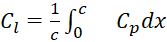(3)

where x is the horizontal coordinate position with origin starting from leading edge.

Airfoil performance takes into account the Reynolds number, Re, which is defined as: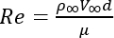(4)

where the new parameter μ is the dynamics viscosity of the fluid.

Here, the overall pressure distribution along the airfoil is measured with 19 small tubes embedded in the wing and attached to a pressure transducer. A Clark Y-14 airfoil is shown in Figure 2. It has a thickness of 14% and is flat on the lower surface from 30% of the chord length to the back.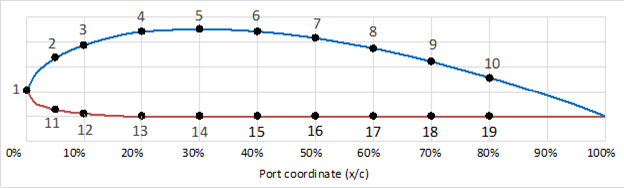Figure 2. Airfoil profile of a Clark Y-14 wing with locations of gage pressure ports.

The gage pressures are measured using a manometer panel with 24 columns filled with liquid oil marked with water inch graduations. The gage pressure reading is determined using the following equation: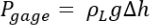(5)

where Δh is the height difference of the manometer with reference to free-stream pressure, ρL is the density of the liquid in the manometer, and g is the acceleration due to gravity.

Once the pressure distribution is obtained, the non-dimensional lift coefficient, Cl, can be numerically determined to evaluate Equation 3: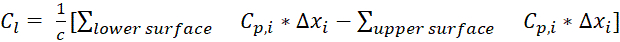(6)

where Δxi is the increment between 2 adjacent ports.

### Procedure

1. Remove top cover of test section to install the Clark Y-14 model (chord length, c = 3.5 in). The test section should be 1 ft x 1 ft and the wind tunnel should be able to sustain a maximum airspeed of 140 mph.
2. Mount the aluminum Clark Y-14 model on the turntable inside the test section so that port #1 is facing upstream. Replace top cover. Note that the model is touching both the floor and the ceiling of the wind tunnel test section so no 3D flow around the airfoil develops.
3. Connect the 19 pressure tubes labeled 1 - 19 to the corresponding ports of the manometer panel, respectively. The ports on the Clark Y-14 model are located as follows: port 1: x/c = 0 (right on leading edge), ports 2 and 11: x/c = 5%, ports 3 and 12: x/c = 10%, ports 4 and 13: x/c = 20%, ports 5 and 14: x/c = 30% ports 6 and 15: x/c = 40%, ports 7 and 16: x/c = 50%, ports 8 and 17: x/c = 60%, ports 9 and 18: x/c = 70%, and ports 10 and 19: x/c = 80% (Figure 2). The manometer panel should have 24 columns filled with colored oil and marked with water inch graduations.
4. Rotate the turntable so that the angle of attack is 0°.
5. Run the wind tunnel at 90 mph, and record all 19 pressure measurements by reading the manometer.
6. Repeat steps 4 and 5 for angles of attack of 4 and 8°.

An airfoil is a two-dimensional wing section that generates lift in an aircraft. Airfoils come in many geometries, but they are all described by the same features. The leading edge is the point at the front of the airfoil with maximum curvature. And similarly, the trailing edge is the point of maximum curvature at the back of the airfoil.

The chord line is a straight line connecting the leading and trailing edges. The chord length, c, is the length of this chord line and is used to describe the dimensions in other directions as percentages of the chord length.

Here, we will focus on the Clark Y-14 airfoil, which has a thickness of 14% chord length and is flat on the lower surface from 30% chord back to the trailing edge. At various angles of attack, the airfoil generates lower pressures on the upper surface and higher pressures on the bottom surface with respect to the approaching air pressure.

According to Bernoulli's Principle, this pressure difference is a result of differences in velocity between the upper and lower regions of the airfoil, which are caused by air molecules interacting with the curved surfaces. The lower pressure region on the upper surface has a higher velocity than the higher pressure region on the lower surface.

If the shear forces parallel to the surface of the airfoil are neglected, then the overall pressure force is what generates lift. We can define the pressure coefficient, Cp, for an arbitrary point on the airfoil using this relationship.The pressure coefficient is a non-dimensional number, which describes the relative pressures throughout a flow field. P is the absolute pressure, P infinity is the free-stream pressure, and rho infinity and V infinity are the free stream density and velocity, respectively.

Except for leading edge locations, the pressure force directions determined by Cp, approximately point upward in the same direction as lift at low angles of attack. Thus, we can calculate a non-dimensional lift coefficient, CL, which relates generated lift to the fluid flow around the object using this relationship. Here, c is the chord length and x is the horizontal coordinate position with zero as the leading edge.

In this experiment, we will analyze the pressure distribution on the surface of an airfoil, which has 19 pressure taps on its surface. Each of the pressure readings are measured using a liquid manometer. You will measure the pressure distribution and lift by subjecting the airfoil to airflow in a wind tunnel at various angles of attack.

For this experiment, you will use an aerodynamic wind tunnel with a test section of 1 ft by 1 ft and a maximum operating air speed of 140 mph. The model airfoil is an aluminum Clark Y-14 airfoil with 19 built-in ports for pressure tubes. The locations of the pressure ports are shown here. The port coordinate is determined by dividing the location of the port by the chord length. The pressure ports are connected to a manometer panel filled with colored oil but marked as water-inch graduations.

To begin, remove the top cover of the test section and install the airfoil vertically on the turntable, making sure that port number one is facing upstream. Replace the top cover of the test section. Note that the airfoil model is touching both the floor and ceiling of the wind tunnel test section in order to make sure there is no 3D flow developed around the airfoil.

Connect the 19 labeled pressure tubes to the corresponding ports of the manometer. Now rotate the turntable for the angle of attack to be zero. Then, turn on the wind tunnel and set the wind speed to 90 mph. Record all 19 manometer height readings in your notebook.

Now turn the wind tunnel off and adjust the angle of attack to 4°. Then, turn the wind tunnel back on with the wind speed at 90 mph and record the manometer readings for each of the 19 pressure ports. Finally, repeat the measurement at 90 mph for an angle of attack of 8°. Like before, record all manometer readings.

Now let's take a look at how to analyze the data. First, determine the gage pressure for each of the manometer height readings using this relationship, where delta h is the height reading recorded in your notebook, rho L is the density of the oil, and g is gravitational acceleration. Next, calculate the non-dimensional pressure coefficient, Cp, for each port on the airfoil.

The pressure coefficient is calculated as shown using the free-stream density, the free-stream velocity and the gage pressure. Let's plot the negative pressure coefficient versus the port coordinate. First, for an angle of attack equal to zero, we plot negative Cp instead of positive Cp on the y-axis in order for the plot to be more visually intuitive. Thus, the top trace conveys the negative pressures on the upper surface of the airfoil, and the bottom trace conveys the positive pressures on the lower surface.

From the plot, we can see that the pressure changes drastically right after the leading edge. The pressure reaches its minimum value around 5 to 15% chord after the leading edge. As a result, about half of the lift is generated in the first 1/4 chord region of the airfoil. Looking at all three angles of attack, we observe a similar pressure change after the leading edge.

Additionally, in all three cases, the upper surface contributes more lift than the lower surface. As a result, it is critical to maintain a clean and rigid surface on the top of the wing. This is why most airplanes are cleared of any objects on the top of the wing.

Before stall occurs, increasing the angle of attack results in higher pressure differences between the bottom and top surfaces of the airfoil, thus generating higher lift. We can calculate the lift coefficient for each angle of attack using the relationship shown here. The lift coefficient relates the generated lift to the pressure distribution on the airfoil and as expected is higher for higher angles of attack.

In summary, we learned how pressure differences along an airfoil generate lift in an aircraft. We then measured the pressure distribution along the surface of a Clark Y-14 airfoil subjected to airflow at various angles of attack and calculated the lift coefficients.

### Results

The results of the lab are shown in Table 1 and Table 2. The data is plotted in Figure 3, which shows the pressure coefficient, Cp, versus the pressure port coordinate, x/c, for angles of attack at 0, 4, and 8°. To be more visually intuitive, the negative Cp values are plotted above the horizontal axis. This is to show that the upper surface (the top line of the chart) is mostly negative pressures and the lower surface (the bottom line of the chart) is mostly positive pressures.

From Figure 3, the pressure significantly changes immediately after the leading edge: the pressure reaches its minimum (or maximum absolute) values at approximately 5% - 15% chord length. As a result, half of the lift is generated in the first quarter of the chord length of the airfoil. Additionally, the upper surface contributes more lift than the lower surface: in all 3 cases, the upper surface contributed to about 70 - 80% of the total lift. Therefore, it is critical to maintain a clean and rigid surface on the top of the wing.

 Pressure port # Ports coordinates x/c Pgage from manometer (in. water) Calculated pressure coefficient Cp 1 0.0 3.7 1.00 2 0.05 -1.2 -0.67 3 0.10 -3.0 -1.00 4 0.2 -3.9 -0.79 5 0.3 -3.4 -0.57 6 0.4 -3.0 -0.55 7 0.5 -2.5 -0.53 8 0.6 -2.3 -0.33 9 0.7 -1.5 -0.31 10 0.8 -0.8 -0.20 11 0.05 -0.7 1.00 12 0.10 -0.6 0.29 13 0.2 -0.3 0.28 14 0.3 -0.2 0.24 15 0.4 0.1 0.22 16 0.5 0.1 0.21 17 0.6 0.2 0.21 18 0.7 0.2 0.21 19 0.8 0.3 0.21

Table 1. Experimental results at zero angle of attack.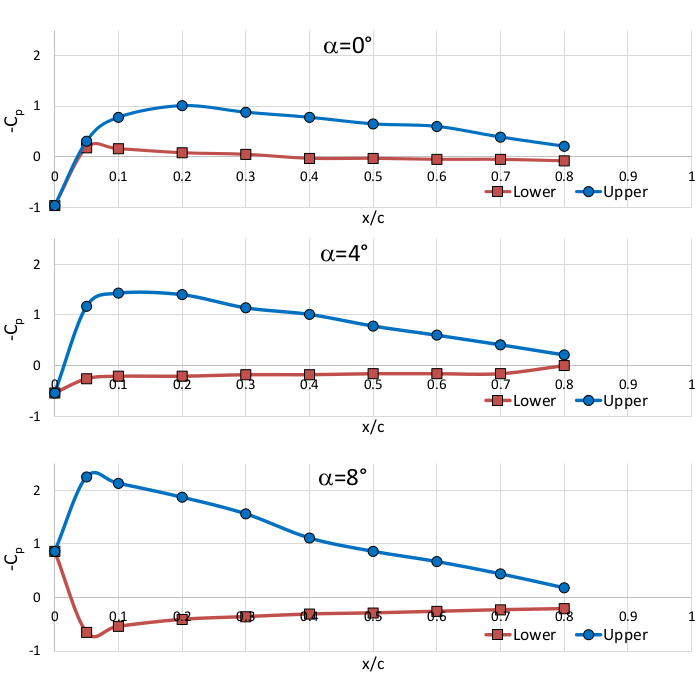Figure 3. Pressure coefficient distribution, Cp, vs position coordinate, x/c.

 Angle of attack Lift coefficient cl 0° 0.53 4° 0.89 8° 1.29

Table 2. Lift coefficient, cl, estimated based on pressure distribution (Re = 2.34 x 105).

 Parameters Values Air density ρ 0.00230 slug/ft3 Water density ρL 1.935 slug/ft3 Gravitational acceleration g 32.17 ft/s2 Viscosity m 3.79 x 10-7 lbf*s/ft2 Free-stream airspeed V∞ 90 mph Reynolds number Re 2.34 x 105 Chord length c 3.5 in

Table 3. Parameters used for calculations.

### Applications and Summary

Pressure distributions on airfoils are directly related to lift generation and important information to characterize the performance of airfoils. Airfoil designers manipulate pressure distributions to acquire desired characteristics of airfoils. As such, pressure distribution information is the foundation of aerodynamics analysis during aircraft development.

In this experiment, the pressure distribution of Clark Y-14 was investigated in a wind tunnel and the 19 ports of pressure measurement were made to find the pressure distribution along the upper and lower surface of the airfoil. The lift coefficient is also calculated reasonably from pressure distribution data.X